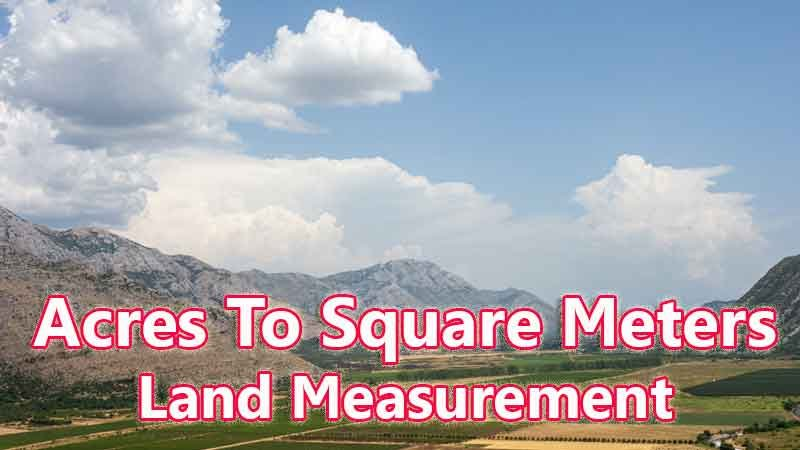# Acres To Square Meters Converter

Acres is a traditional land unit used to measure the land Area standardly. Here we are using the acres to square meters unit value conversion. And acres also comes under these land area units like Gonda, Kani, and square meters. In West Bengal, One acres is directly equivalent to 4046.86 square meters.

Input Here

acres

Output

square meters

1 acres = 4046.86 square meters
Rounded Value Is (1 acres = 33 square meters)

## Measurement

acres means - a unit of land measurement. This tool provides great calculation over acres to square meters, 1 acres to square meters, 1 acres in square meters, 1 acres equal to and etc. To convert acres to square meters, then multiply the unit value by 4046.86. Then, 1 acres * 4046.86 = 4046.86 square meters. Hence, 1 acres is equal to 4046.86 square meters.

square meters also comes under this land measurement unit and we are comparing values between acres traditional unit with other side land measurement unit.

## Formula For acres to square meters Conversion(acres to square meters formula)

The base formula for this acres to square meters converter is

square meters = acres * 4046.86

## How many square meters in a acres?

Step 1: To Convert 1 acres to square meters

Step 2: Applying formula square meters = acres * 4046.86, (i.e) multiply the unit value by 4046.86.

Step 3: Then, square meters = 1 * 4046.86 = 4046.86.

Step 4: Hence, 1 acres is equal to 4046.86 square meters.Some acres to square meters Conversion Chart for your reference:

 1 acres = 4046.86 square meters 2 acres = 8093.72 square meters 3 acres = 12140.58 square meters 4 acres = 16187.44 square meters 5 acres = 20234.3 square meters 6 acres = 24281.16 square meters 7 acres = 28328.02 square meters 8 acres = 32374.88 square meters 9 acres = 36421.74 square meters 10 acres = 40468.6 square meters

The acres to square meters conversion chart is above listed for your reference. This chart, however, represents the simple math calculation involved in the acres to square meters convertion online.

For Example: How much is 1 acres to square meters

Solution:

= (acres * 4046.86)

= (1 x 4046.86)

= 4046.86 square meters

For Example: How much is 120 acres in square meters

Solution:

= (acres * 4046.86)

= (120 x 4046.86)

= 485623.2 square meters

This formula provides an instant answer for all your questions / People also search:

• 1 acres to square meters
• 1 acres is equal to how many square meters
• Formula to convert acres to square meters online
• How to convert acres to square meters?
• How much square meters is equal to a one acres?
• How many square meters in acres?
• 1 acres equal to square meters